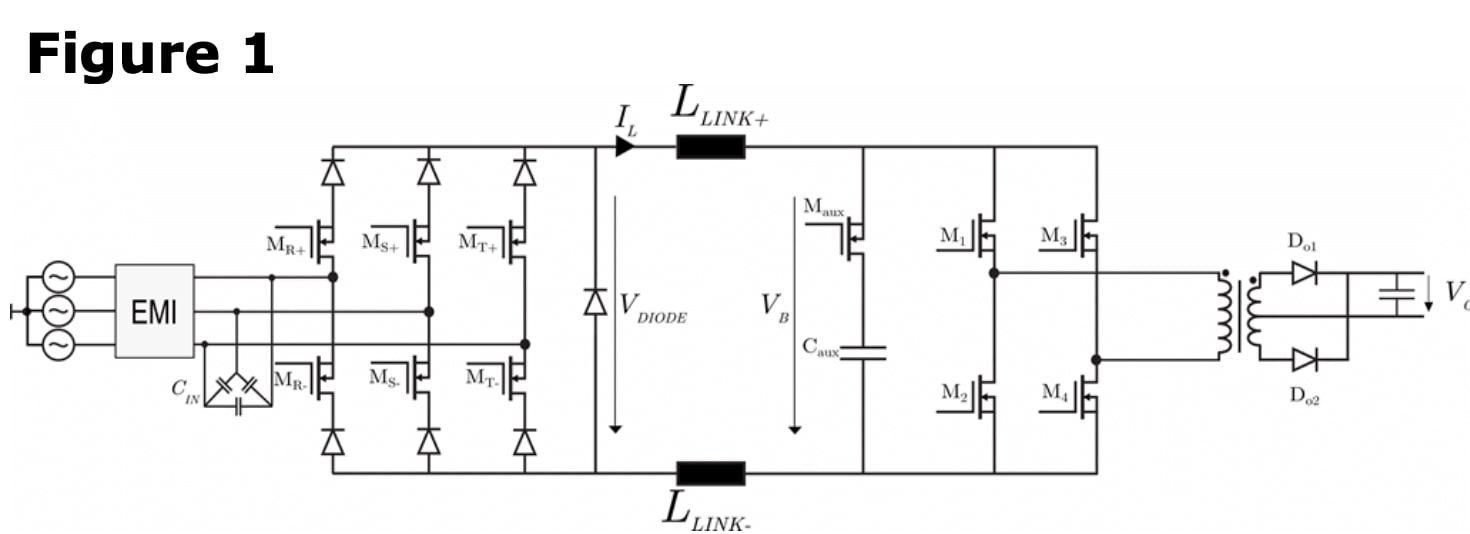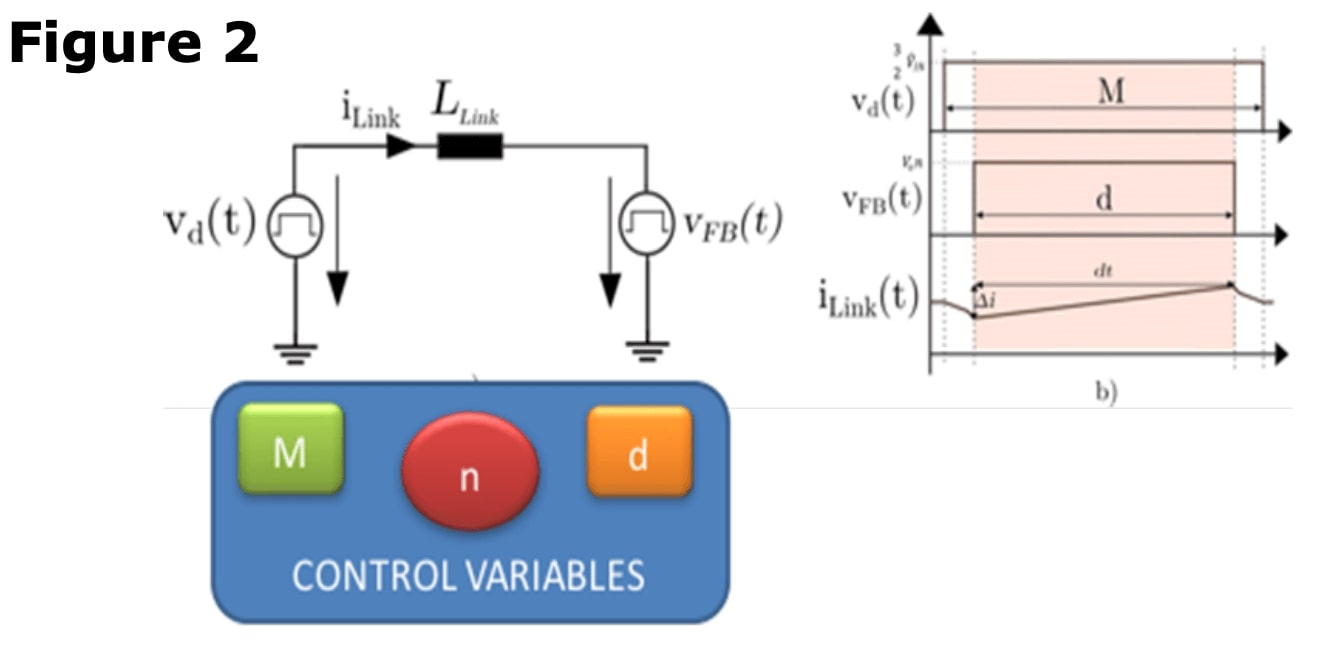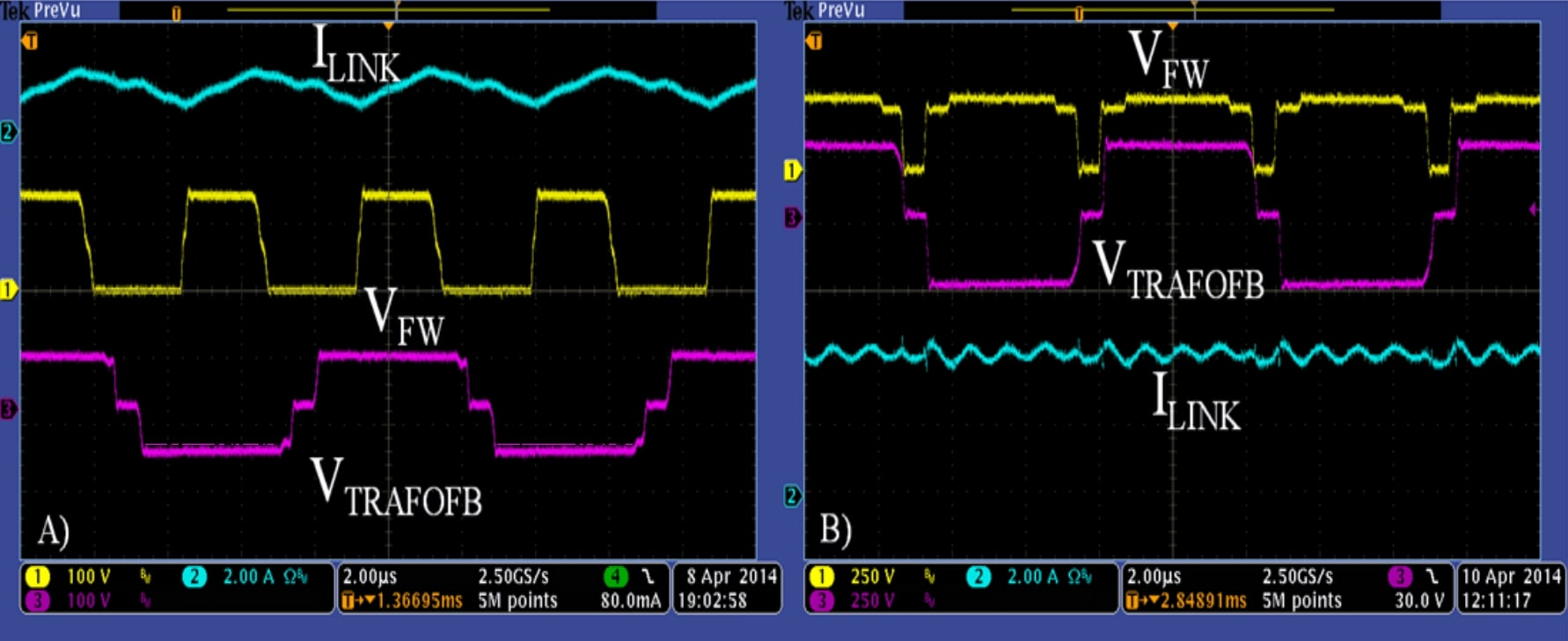# Effective way to reduce inductor's size by synchronizing both power stages

The size of the magnetics is one of the actual bottlenecks in the power electronics industry. There are several strategies for decreasing the size of the magnetics, increasing the switching frequency, integrated magnetics, flux cancellation...

In this application note, it is presented one strategy based on synchronizing both power stages, a voltage fed topology in cascade with a current fed circuit.

## Control Strategy Model

It is very common to need several stages in actual power architectures due to restricted specifications as isolation or conversion ratios. In the cases where a voltage-fed converter (Buck, Classic Full-Bridge, Forward) supplies a current fed converter (Boost, Current Fed Full-Bridge), both systems share an inductor. A specific example of this situation is discussed in this app note. The circuit presented in Figure 1, presents a three-phase Buck type rectifier and a current Fed Full-Bridge. Figure 2 shows the simplified model, considering each stage as a voltage source controlled by parameters M and d.The first stage establishes a voltage on one side of the output inductor and the second converter another. What it is presented in this App Note is the idea of synchronizing both pulsating voltages and adding the turns ratio of the transformer as a variable to reduce the voltage increment in the inductor, reducing the current ripple and consequently, the size. With this strategy, we are able to decrease the inductor size by 30%. The only fact of synchronizing both stages is to reduce the current ripple and losses related. If additionally, with the turn ratio, the voltage amplitude can be similar, the reduction is even better.### Experimental Results

The experimental waveforms show how both voltages are synchronized and the resultant ripple depends on the pulse width of both values. Since the circuit described is an AC/DC rectifier, the voltage amplitude, and the pulse width depend on the input voltage evolution. Therefore, the current ripple will present a minimum when both values are almost equal, as shows the Figure 3 (left). Nevertheless, when the voltages amplitude and the pulse width are not equal, the ripple is increased. The trade-off for the operating point for the minimum ripple will depend on the designer.### Conclusions

In conclusion, control strategies for reducing the ripple are very convenient for reducing the inductor size, especially in several power stages strategies.

Do you want the full version?